# Unit 3 Topic 5 Chemical Reactions Chemical reactions

• Slides: 20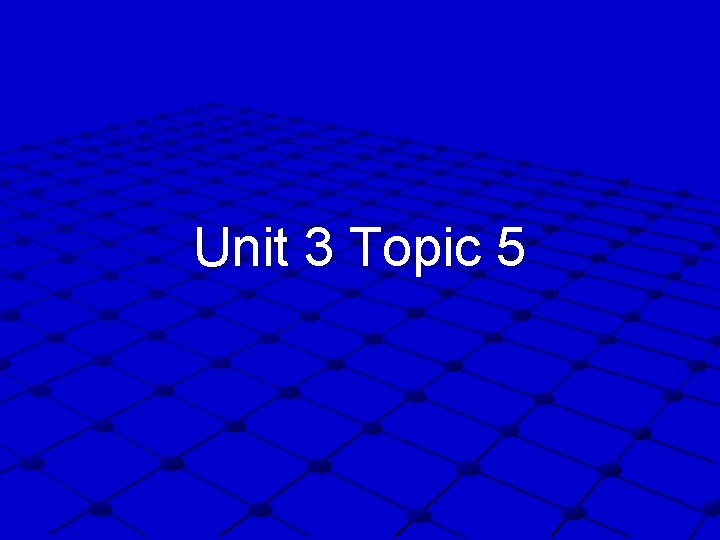Unit 3 Topic 5Chemical Reactions Chemical reactions can be described by writing balanced chemical equations A balanced chemical equation shows the formulas of both the reactants (what you start with) and the products (what was made), the mole ratio of how they interact and their physical states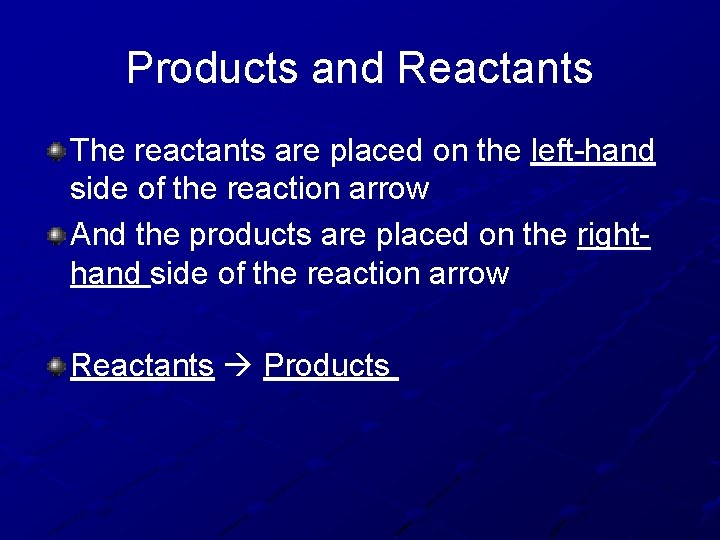Products and Reactants The reactants are placed on the left-hand side of the reaction arrow And the products are placed on the righthand side of the reaction arrow Reactants ProductsBalancing Balanced chemical equations must have the same number of each type of element on each side of the reaction arrow Mass is conserved because the number of atoms is conserved (the same on both sides) It is extremely important to be able to correctly interpret chemical equations.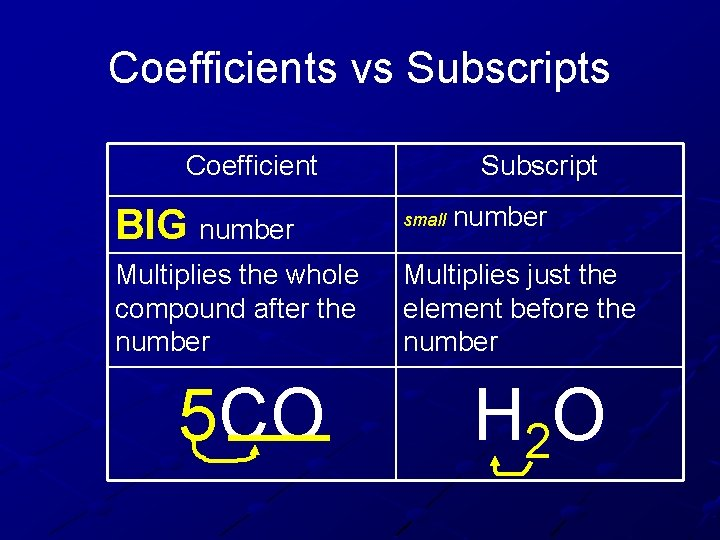Coefficients vs Subscripts Coefficient Subscript number BIG number small Multiplies the whole compound after the number Multiplies just the element before the number 5 CO H 2 OWhat does this mean? This reaction shows 2 compounds combining to form 2 new compounds, however their masses did not change! The same atoms are all there, they have just rearranged themselves. Source: http: //www. tea. state. tx. us The amount of matter we start with is the same amount of matter we finish with.Ca. Cl 2 + Na 2 SO 4 Ca. SO 4 + Na. ClCa. Cl 2 + Na 2 SO 4 Ca. SO 4 + Na. Cl Ca. Cl 2 Ca Cl N S O Na 2 SO 4 Ca. SO 4 Na. Cl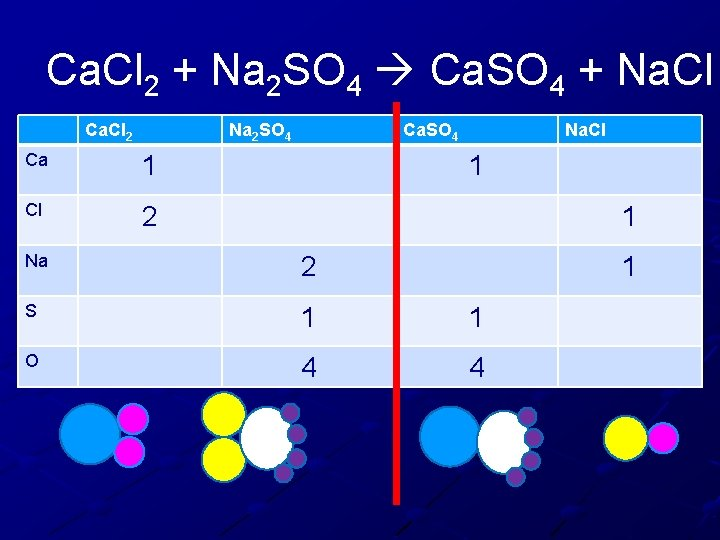Ca. Cl 2 + Na 2 SO 4 Ca. SO 4 + Na. Cl Ca. Cl 2 Na 2 SO 4 Ca 1 Cl 2 Ca. SO 4 Na. Cl 1 1 Na 2 S 1 1 O 4 4 1Ca. Cl 2 + Na 2 SO 4 Ca. SO 4 + Na. Cl 1. 2. 3. 4. 5. 6. 7. 8. 9. Calcium is balanced Need more Chlorine on the right Add another Na. Cl Recheck Calcium is balanced Chlorine is balanced Sodium is balanced Sulfur is balanced Oxygen is balanced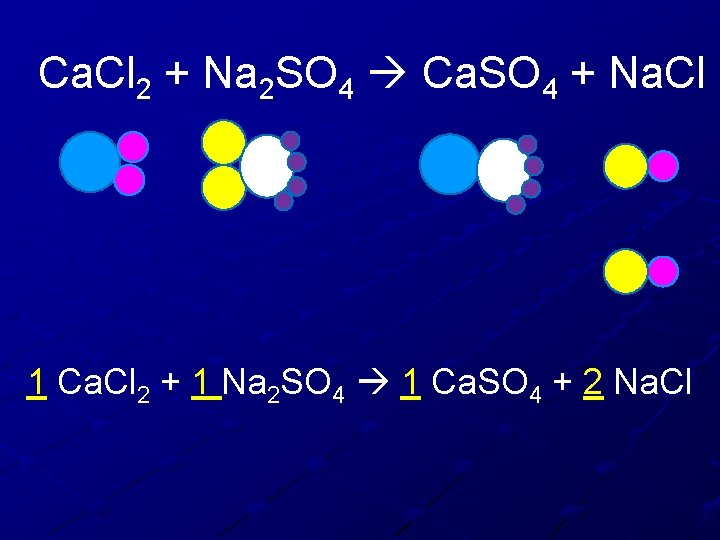Ca. Cl 2 + Na 2 SO 4 Ca. SO 4 + Na. Cl 1 Ca. Cl 2 + 1 Na 2 SO 4 1 Ca. SO 4 + 2 Na. ClH 2 O(l) + Fe(s) Fe 3 O 4(s) + H 2 (g) H O Fe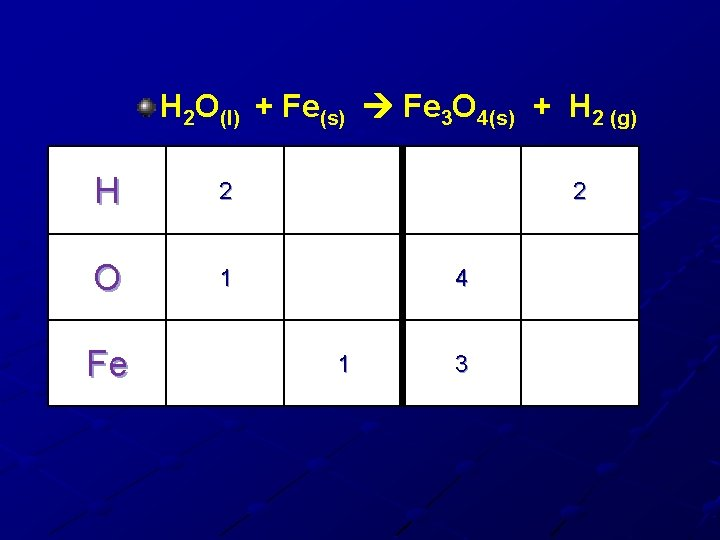H 2 O(l) + Fe(s) Fe 3 O 4(s) + H 2 (g) H 2 O 1 Fe 2 4 1 3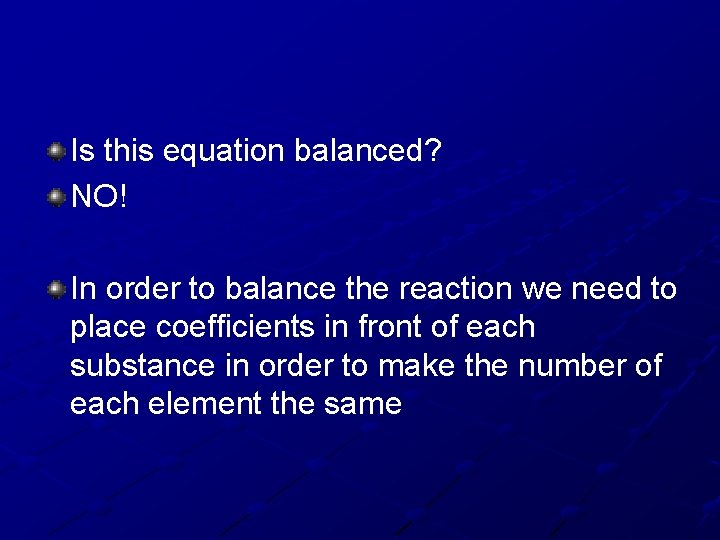Is this equation balanced? NO! In order to balance the reaction we need to place coefficients in front of each substance in order to make the number of each element the sameCoefficients H 2 O(l) + Fe(s) Fe 3 O 4(g) + H 2 (g) H H O Fe Fe Are the Hydrogen’s balanced? Are the Oxygens’s balanced? O H O O YES, both sides have 2 NO, left side has 1 right side has 4 WHAT ARE WE GOING TO DO ABOUT IT?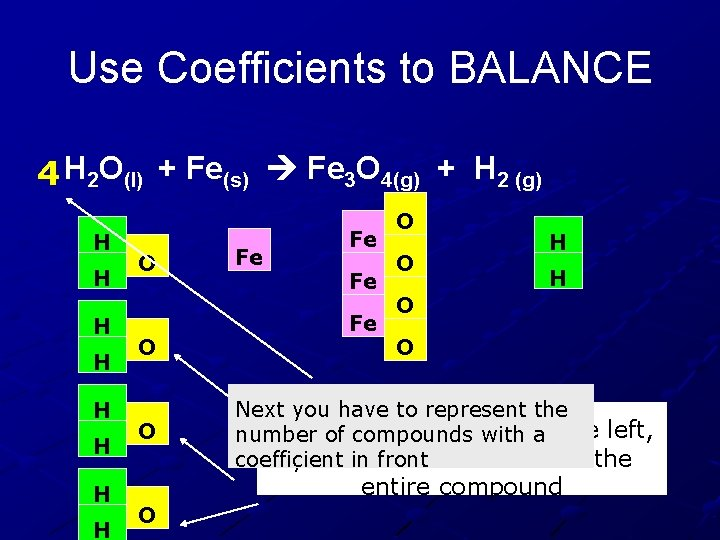Use Coefficients to BALANCE 4 H 2 O(l) + Fe(s) Fe 3 O 4(g) + H 2 (g) H H H H O O O Fe Fe O O H H O O Next you have to represent the To add more Oxygen number of compounds with to a the left, you have to add more of the coefficient in front entire compound O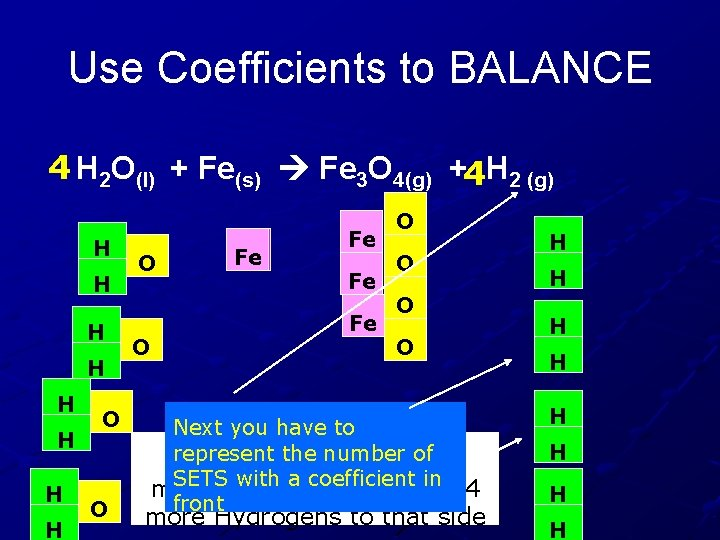Use Coefficients to BALANCE 4 H 2 O(l) + Fe(s) Fe 3 O 4(g) +4 H 2 (g) H H H H O O Fe Fe O O Next you have to represent the number need of Now the Hydrogens SETS with a coefficient in more on the right, so add 4 front more Hydrogens to that side H H H HUse Coefficients to BALANCE 4 H 2 O(l) + 3 Fe(s) Fe 3 O 4(g) +4 H 2 (g) H H H H O O O Fe Fe O O H H H Then use a coeffient to Next, the irons need toofbe represent the number balanced out by adding 2 iron SETS more to the left side. H H HWhen you finish with the Legos Complete Page 11 and show Mrs. Liller for credit. Next complete pages 12 and 13. What you don’t finish do for homework. Balancing Quiz Friday 11/14 I need to see page 7 and 8 from: Derrick Hevert James William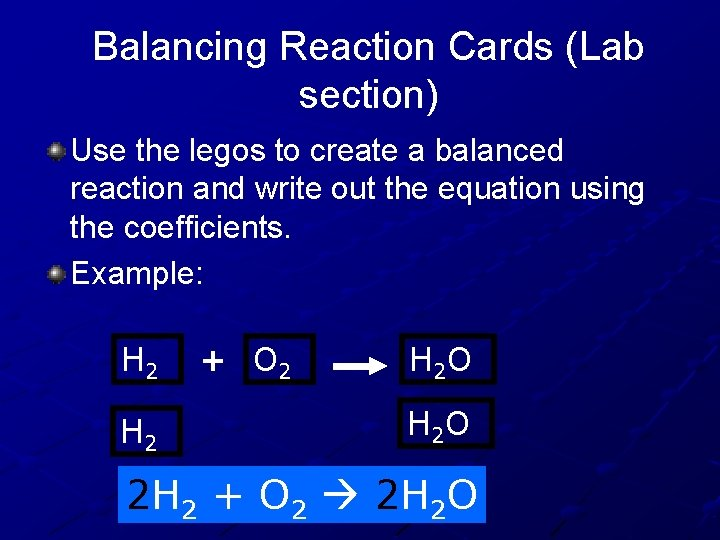Balancing Reaction Cards (Lab section) Use the legos to create a balanced reaction and write out the equation using the coefficients. Example: H 2 + O 2 H 2 O 2 H 2 + O 2 2 H 2 O